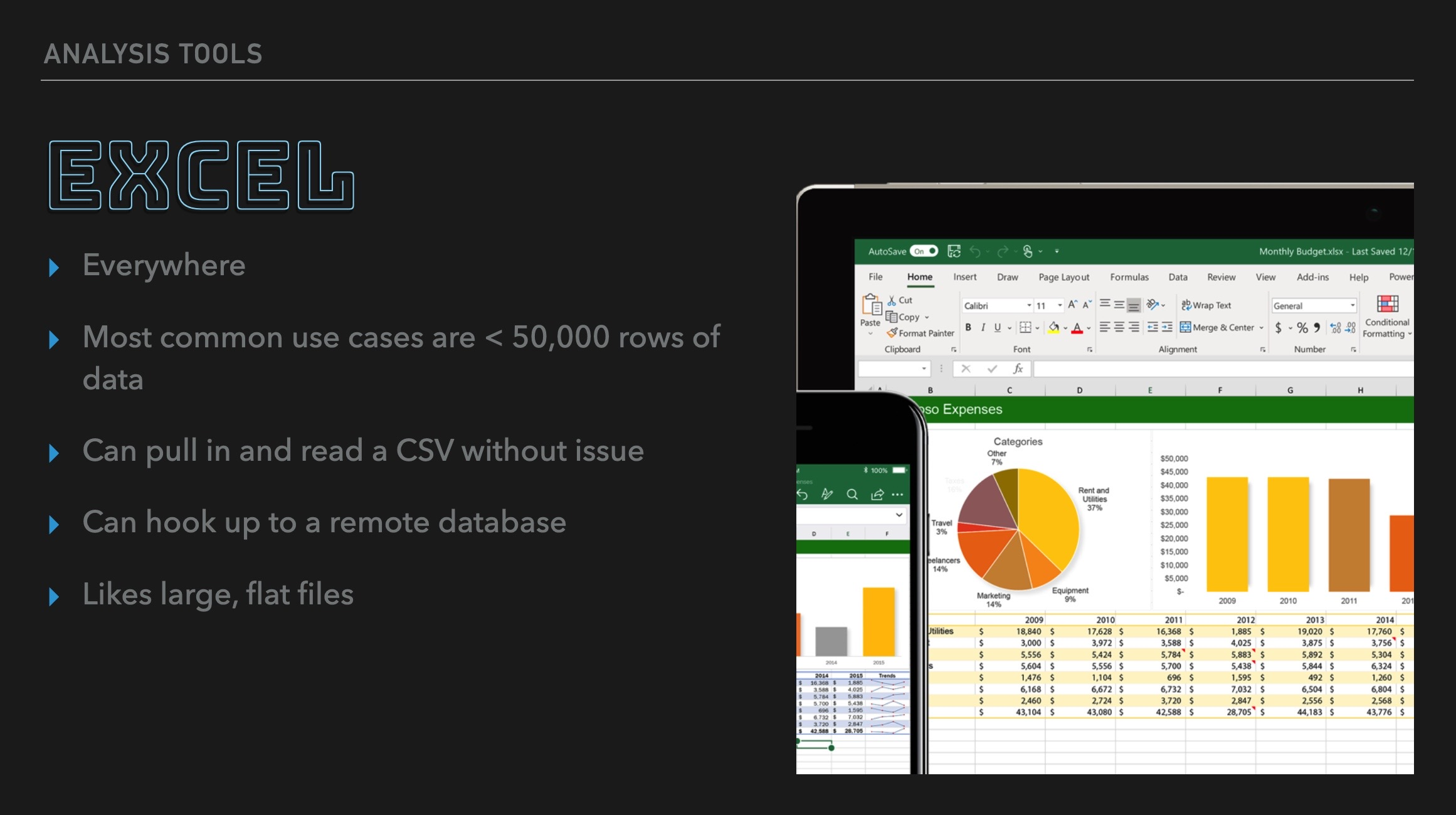👋🏼

We've been asked to assemble two queries for export: the chemical data gathered per flyby and the chemical data found over the entirety of the Cassini mission. We can do that using these queries:

``````set search_path='enceladus';
select flybys.name as flyby,
inms.date,
inms.source,
chemistry.name as compound,
chemistry.formula,
sum(inms.high_sensitivity_count) as sum_high,
sum(inms.low_sensitivity_count) as sum_low
from flybys
inner join inms on flyby_id = flybys.id
inner join chemistry on chemistry.molecular_weight = inms.mass
group by flybys.name, inms.date, inms.source, chemistry.name, chemistry.formula;
``````

To speed things up (and uncramp our fingers) we'll create a materialized view. Notice how I'm dropping the view if it exists and then recreating it directly. This keeps our script idempotent, which is required:

``````set search_path='enceladus';
drop materialized view if exists results_per_flyby;
create materialized view results_per_flyby as
select flybys.name,
flybys.date,
inms.source,
chemistry.name as compound,
chemistry.formula,
sum(inms.high_sensitivity_count) as sum_high,
sum(inms.low_sensitivity_count) as sum_low
from flybys
inner join inms on flyby_id = flybys.id
inner join chemistry on chemistry.molecular_weight = inms.mass
group by flybys.name, flybys.date, inms.source, chemistry.name, chemistry.formula
order by flybys.date;
``````

The overall query is a bit simpler as all we need to do is remove the `flybys` table from the `select`, `join` and `group by` list:

``````select
inms.source,
chemistry.name as compound,
chemistry.formula,
sum(inms.high_sensitivity_count) as sum_high,
sum(inms.low_sensitivity_count) as sum_low
from flybys
inner join inms on flyby_id = flybys.id
inner join chemistry on chemistry.molecular_weight = inms.mass
group by inms.source, chemistry.name, chemistry.formula)
``````